# 第四次作业

1 视频截图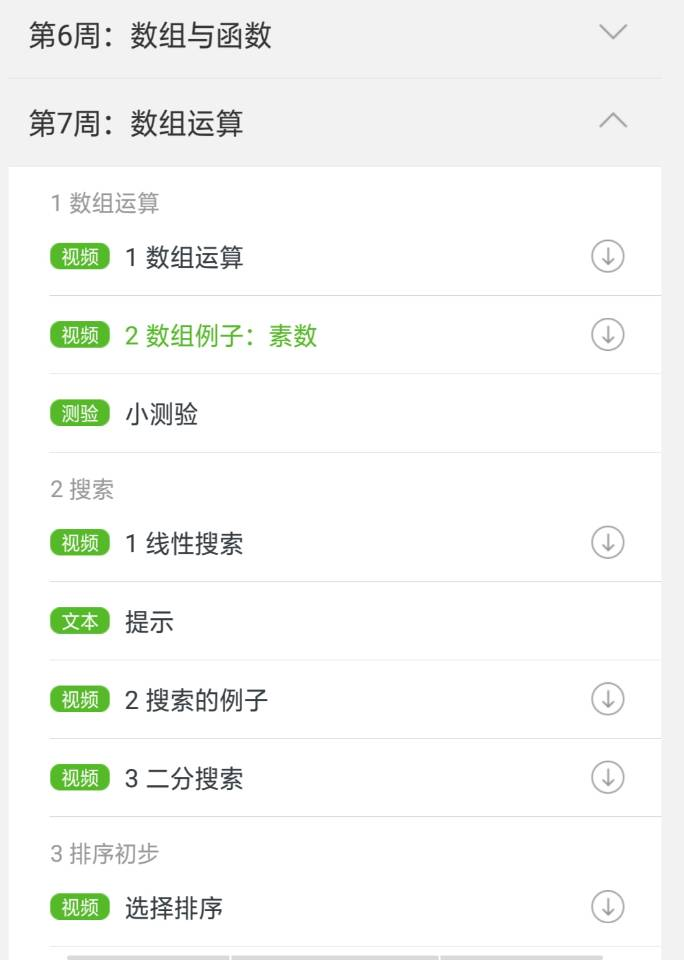2 通过观看视频我明白了

1）数组作为函数参数时，往往必须再用另一个参数来传入数组的大小。

2）判断素数还可以构造素数表来解决。

3）在代码中加大括号可以用来调试。

3.   对于视频中那些关于指针的还不太明白，并且对素数的相关程序掌握的还不够熟练。

### 输出格式:

#include<stdio.h>
int main(void)
{
int n,i,index;
int a;
index = 0;
scanf("%d",&n);
for(i=0;i<n;i++)
{
scanf("%d",&a[i]);
}
for(i=0;i<n;i++)
{
if(a<a[i])
{
a = a[i];
index=i;
}
}
printf("%d %d",a,index);
return 0;
}


解题思路

1 定义n,i,index; n是用户输入的整数的个数。

2 输出最大值与它对应的下标。

3 将最大值与第一个数交换，输出交换后的n个数。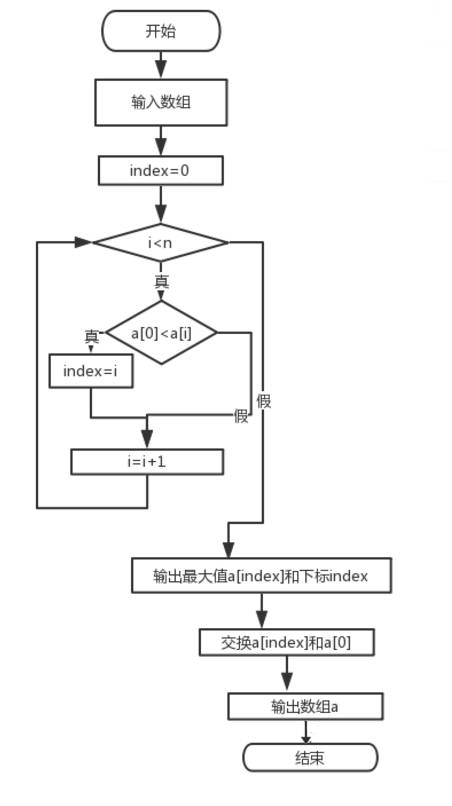总结：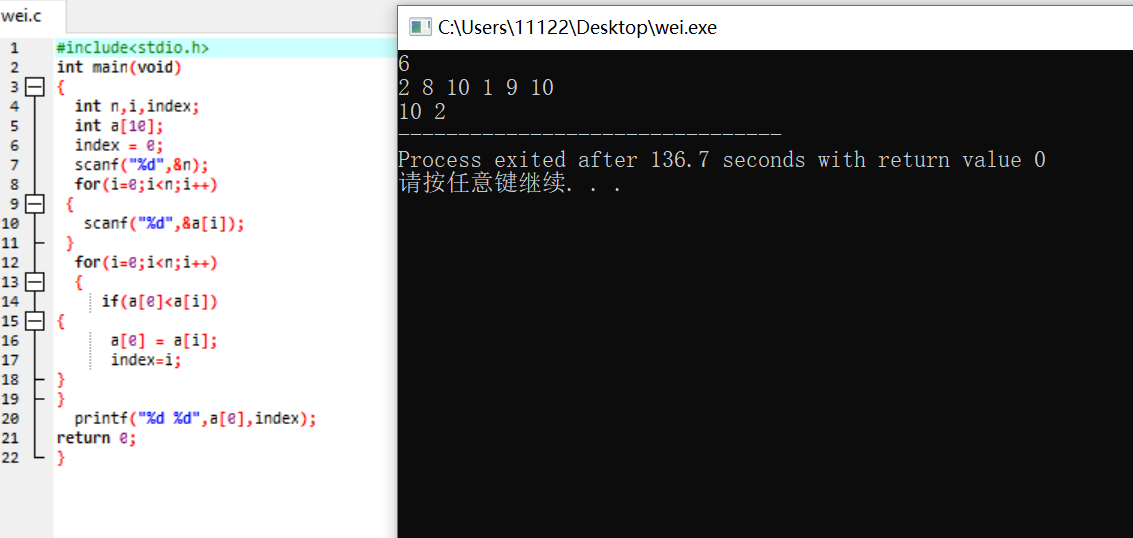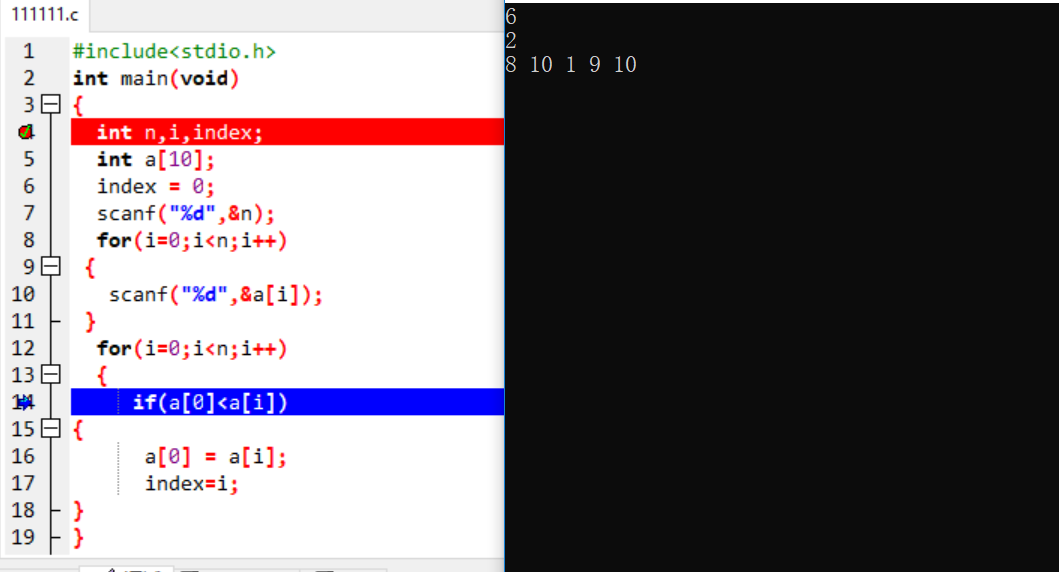### 输出格式:

#include<stdio.h>
int main(void)
{
int i,n;
double average,sum=0;
scanf("%d",&n);
int a[n];
sum=0;
if(n < 1||n>10){printf("Invalid.");}
else{
for(i=0;i<n;i++){
scanf("%d",&a[i]);
sum=sum+a[i];
}
average=sum/n;
printf("%.2f\n",average);
for(i=0;i<n;i++){
if(a[i]>average)
printf("%d ",a[i]);
}
}
printf("\n");
return 0;
}


解题思路

1定义变量 i，n， average，sum；average存放平均值，sum保存数据之和。

2 利用for循环求平均值。

3逐个与平均值比较，输出大于平均值的数。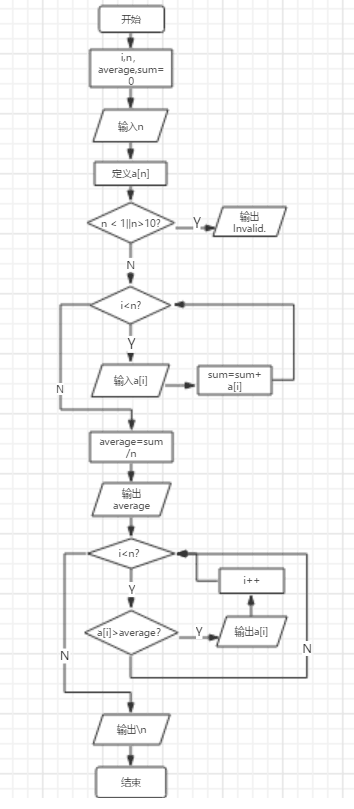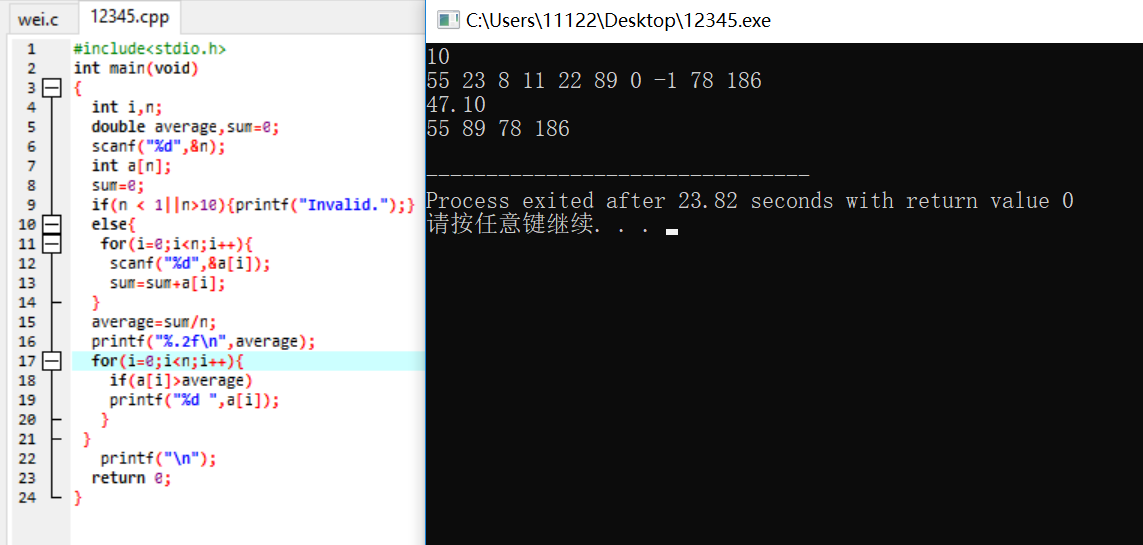调试截图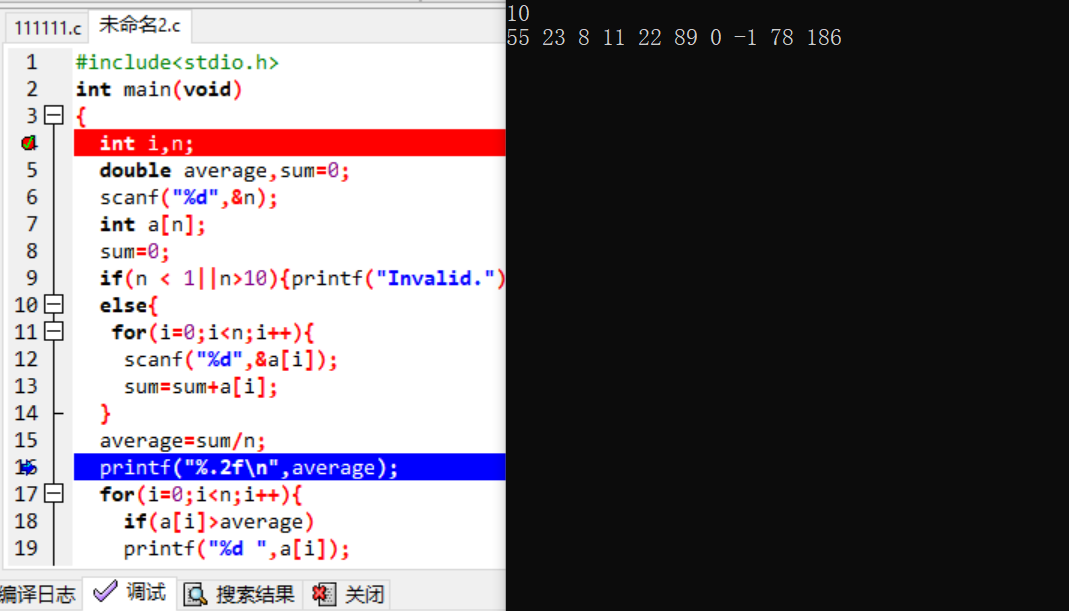学习进度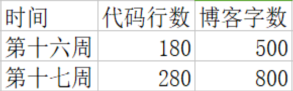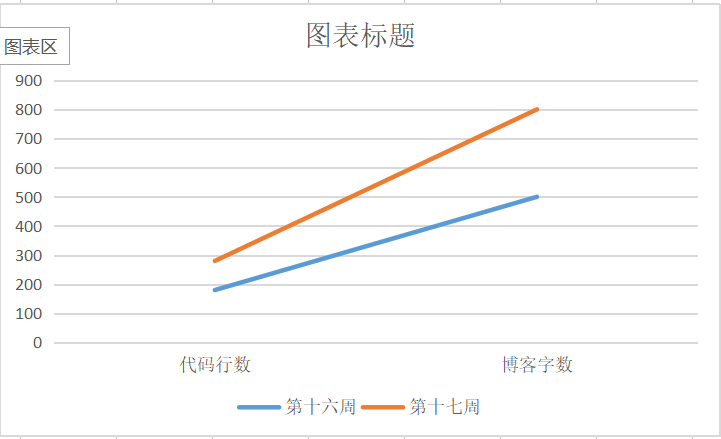posted @ 2018-12-24 19:01  陈雨薇  阅读(2080)  评论(13编辑  收藏  举报